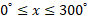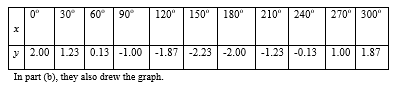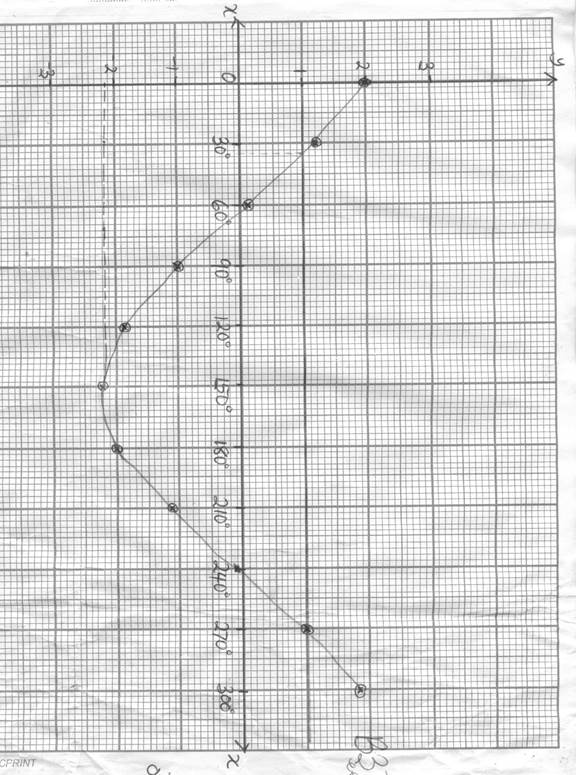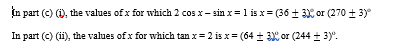General Mathematics Paper 2, WASSCE (PC), 2018

Question 10

1. Copy and complete the following table of values for y = 2 cos x – sin x ,.
 x 0o 30o 60o 90o 120o 150o 180o 210o 240o 270o 300o y 2.00 0.13 -1.87 -2.00 -0.13
1. Using scales of 2 cm to 30o on the x-axis and 2 cm to 1 unit on the y-axis, draw the graph of

y = 2 cos x – sin x for.

1. Use the graph to find the value(s) of x for which:
2. 2 cos x – sin x = 1;
3. tan x = 2.

Observation

The Chief Examiner reported that most Candidates’ were able to complete and fill the table of values as well as drawing the graph. But were unable to answer the questions that followed.
In part (a), they copied and completed that table of values which is